# Common Core: High School - Geometry : Line and Angle Understanding and Applications

## Example Questions

### Example Question #1 : Prove Geometric Theorems: Lines And Angles

What does it mean for two angles to be complementary angles?

Complementary angles are any two angles in a triangle that sum to be 180

Complementary angles are any two angles in a triangle that sum to be 90

Complementary angles are any two angles that sum to be 90

Complementary angles are any two angles that sum to be 180

Complementary angles are any two angles that sum to be 90

Explanation:

The definition of complementary angles is: any two angles that sum to 90.  We most often see these angles as the two angles in a right triangle that are not the right angle.  These two angles do not have to only be in right triangles, however.  Complementary triangles are any pair of angles that add up to be 90.

### Example Question #1 : Prove Geometric Theorems: Lines And Angles

Solve for angle 1.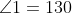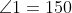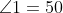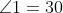### Example Question #2 : Prove Geometric Theorems: Lines And Angles

Lines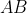and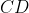are parallel.  Using this information, find the values for angles 1,2,3, and 4.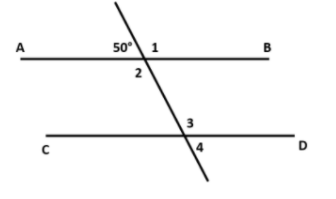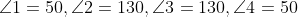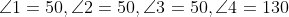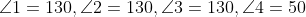Explanation:

We must use the fact that linesandare parallel lines to solve for the missing angles.  We will break it down to solve for each angle one at a time.

Angle 1:

We know that angle 1’s supplementary angle.  Supplementary angles are two angles that add up to 180 degrees.  These two are supplementary angles because they form a straight line and straight lines are always 180 degrees.  So to solve for angle 1 we simply subtract its supplementary angle from 180.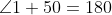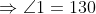Angle 2:

We now know that angle 1 is 130 degrees.  We can either use the fact that angles 1 and 2 are opposite vertical angles to find the value of angle 2 or we can use the fact that angle 2's supplementary angle is the given angle of 50 degrees.  If we use the latter, we would use the same procedure as last time to solve for angle 2.  If we use the fact that angles 1 and 2 are opposite vertical angles, we know that they are congruent.  Since angle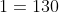then angle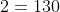.

Angle 3:

To find angle 3 we can use the fact that angles 1 and 3 are corresponding angles and therefore are congruent or we can use the fact that angles 2 and 3 are alternate interior angles and therefore are congruent.  Either method that we use will show that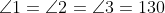.

Angle 4:

To find angle 4 we can use the fact that the given angle of 50 degrees and angle 4 are alternate exterior angles and therefore are congruent, or we can use the fact that angle 3 is angle 4’s supplementary angle.  We know that the given angle and angle 4 are alternate exterior angles so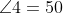.

### Example Question #1 : Line And Angle Understanding And Applications

What are the values of angles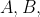and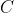?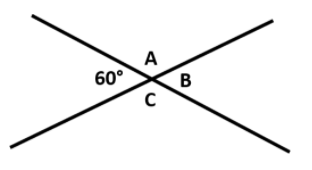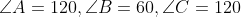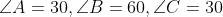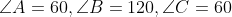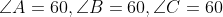Explanation:

We are able to use the relationship of opposite vertical angles to solve this problem.  The given angle and angle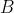are opposite vertical angles and therefore must be congruent.  So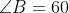is supplementary to both angles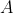andso they must be congruent.andare also opposite vertical angles so they must be congruent in that respect as well.  So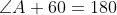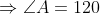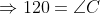### Example Question #5 : Line And Angle Understanding And Applications

True or False: Linesandare parallel.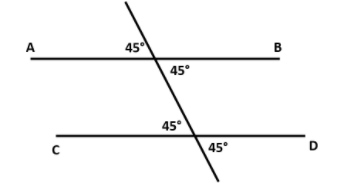False

True

True

Explanation:

We know that linesandare parallel due to the information we get from the angles formed by the transversal line.

There are:

two pairs of congruent vertically opposite angles

two pairs of congruent alternate interior angles

two pairs of congruent alternate exterior angles

two pairs of congruent corresponding angles

Just using any one of these facts is enough proof that linesandare parallel.

### Example Question #121 : Congruence

Are linesandparallel?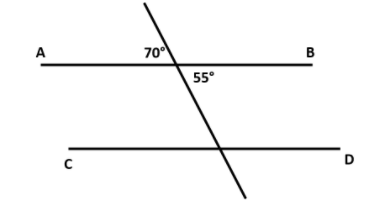No

Yes

No

Explanation:

The angles formed by the transversal line intersecting linesanddoes not form congruent opposite vertical angles.  Therefore these two lines are not congruent.

### Example Question #7 : Line And Angle Understanding And Applications

Which of the following describes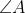and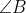?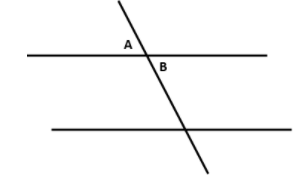These angles are alternate interior angles, therefore they are equal

These angles are alternate exterior angles, therefore they are equal

These angles are corresponding angles, therefore they are equal

These angles are complementary angles, therefore they sum to 90

These angles are alternate exterior angles, therefore they are equal

Explanation:

To answer this question, we must understand the definition of alternate exterior angles.  When a transversal line intersects two parallel lines, 4 exterior angles are formed.  Alternate exterior angles are angles on the outsides of these two parallel lines and opposite of each other.

### Example Question #3 : Prove Geometric Theorems: Lines And Angles

True or False: The following figure shows a line segment.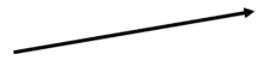False

True

False

Explanation:

The figure shows a ray.  A ray has a single endpoint and the other end extends infinitely.  This is represented by an arrowhead on the end that extends infinitely.

### Example Question #9 : Line And Angle Understanding And Applications

Which of the following describesand?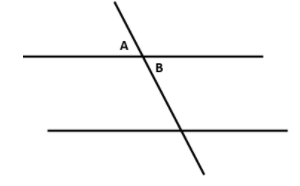These are vertically opposite angles, therefore they are equal

These are vertically opposite angles, there is not enough information to determine any further relation

These are corresponding angles, there is not enough information to determine any further relation

These are corresponding angles, therefore they are equal

These are vertically opposite angles, therefore they are equal

Explanation:

To answer this question, we must understand the definition of vertically opposite angles.  Vertically opposite angles are angles that are formed opposite of each other when two lines intersect.  Vertically opposite angles are always congruent to each other.

### Example Question #1 : Line And Angle Understanding And Applications

Solve for?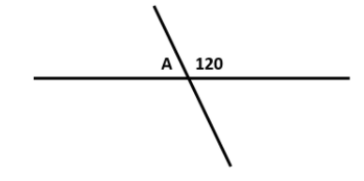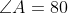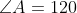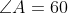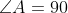Explanation:and the given angle are supplementary angles. This means that they sum up to 180 degrees.

So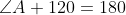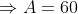### All Common Core: High School - Geometry Resources